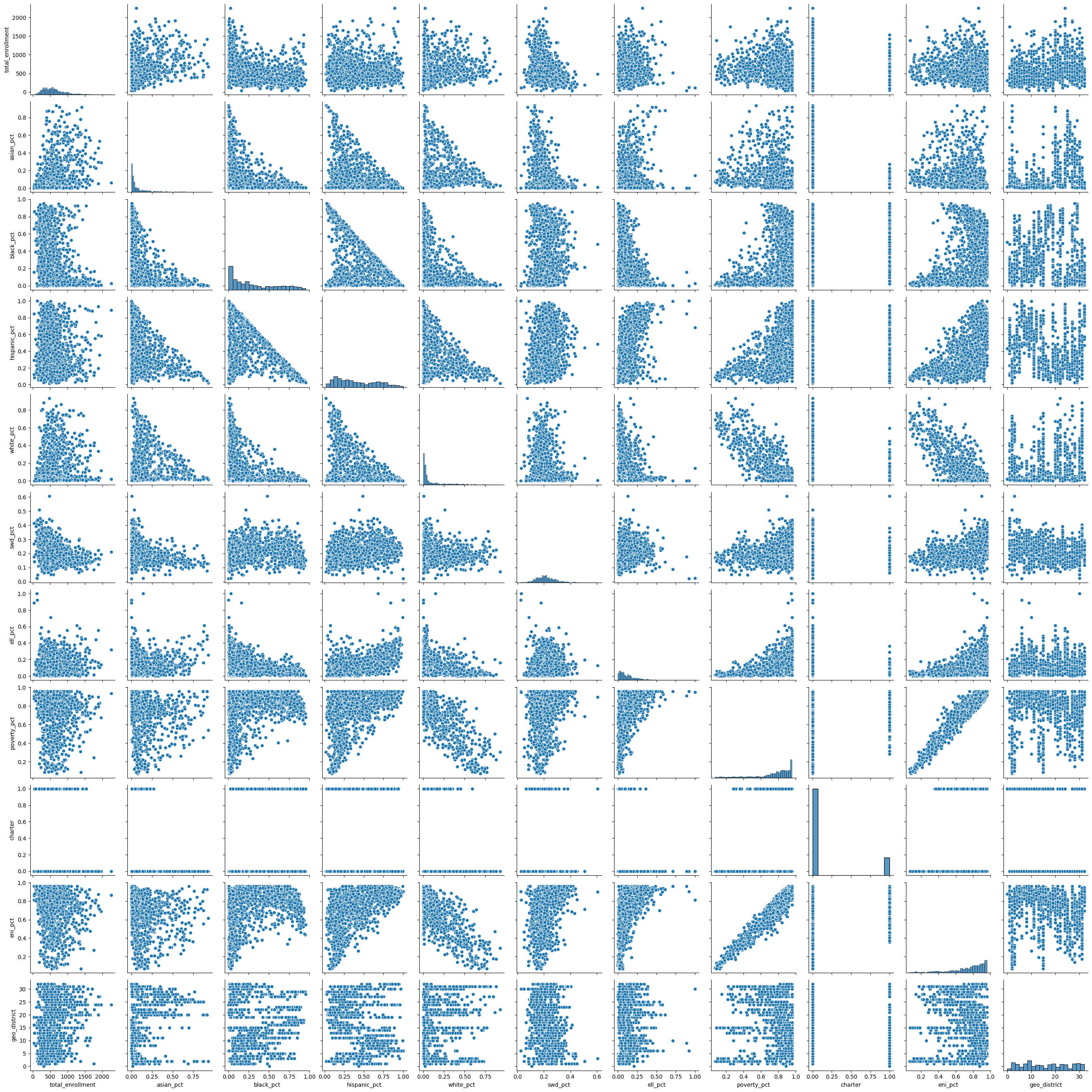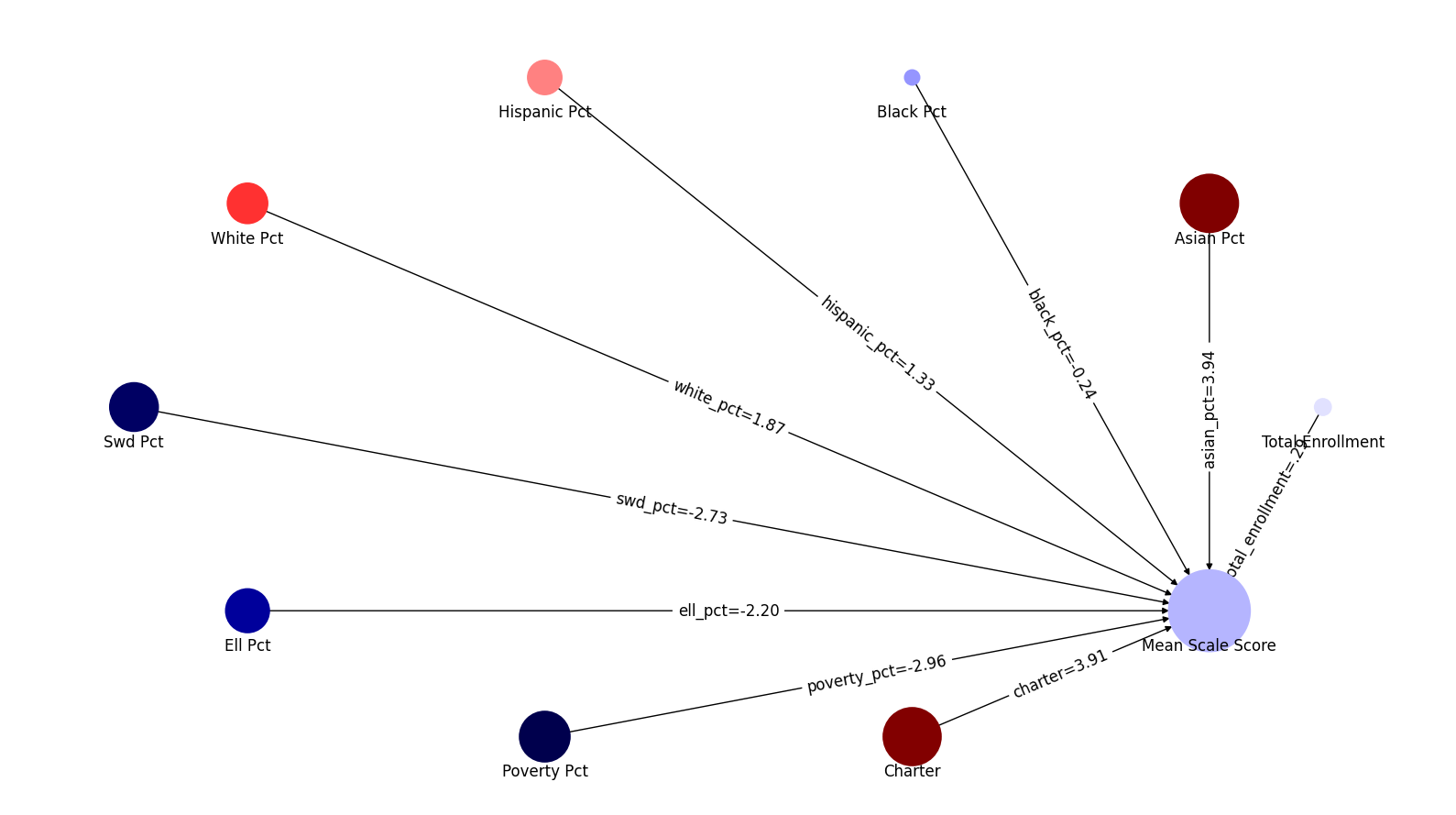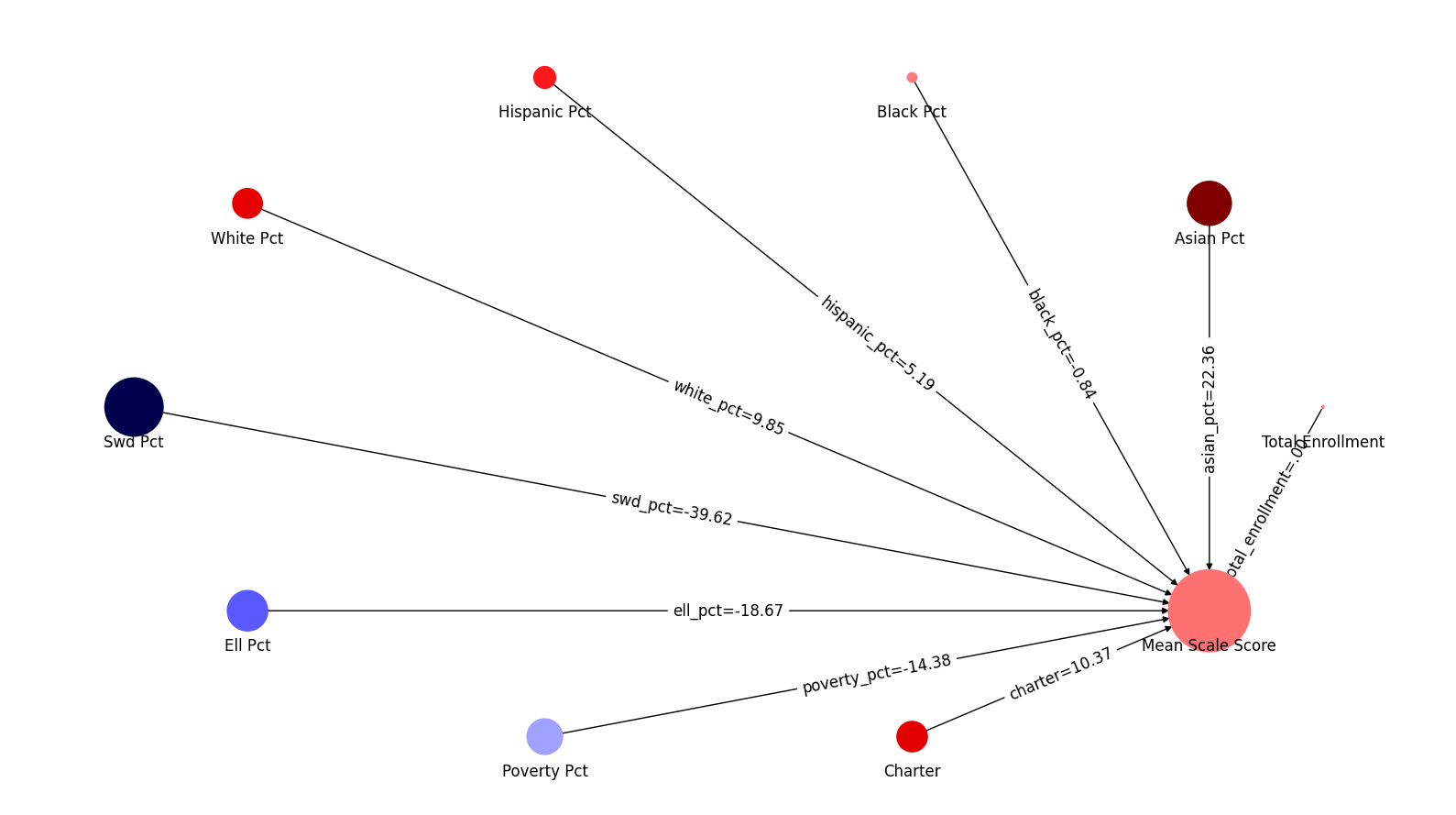# Predictive Statistics#

This notebook uses the sklearn library to run some machine learning predictions using regression analysis. It has examples of both Ordinary Least Square and Partial Least Square Regression.

We look at school demographics to see how well we can use those factors to predict mean_scale_score. We look at the grades 3-8 NYS math exams for the most recent academic year in our data set.

import pandas as pd
import numpy as np

from sklearn.linear_model import LinearRegression
from sklearn.model_selection import train_test_split
from sklearn.metrics import mean_squared_error, r2_score
from sklearn.preprocessing import scale
from sklearn.cross_decomposition import PLSRegression
from sklearn.feature_selection import chi2

import statsmodels.api as sm

import matplotlib.pyplot as plt
import seaborn as sns
import math

from IPython.display import Markdown as md

from nycschools import schools, ui, exams

# load the demographic data and merge it with the math data

math_df = df.merge(math_df, how="inner", on=["dbn", "ay"])
math_df = math_df[math_df["mean_scale_score"].notnull()]

dbn beds district geo_district boro school_name_x short_name ay year total_enrollment ... level_2_pct level_3_n level_3_pct level_4_n level_4_pct level_3_4_n level_3_4_pct test_year charter school_name_y
0 01M015 310100010015 1 1 Manhattan P.S. 015 Roberto Clemente PS 15 2016 2016-17 178 ... 0.310345 7.0 0.241379 5.0 0.172414 12.0 0.413793 2017 0 NaN
1 01M015 310100010015 1 1 Manhattan P.S. 015 Roberto Clemente PS 15 2016 2016-17 178 ... 0.347826 8.0 0.347826 1.0 0.043478 9.0 0.391304 2017 0 NaN
2 01M015 310100010015 1 1 Manhattan P.S. 015 Roberto Clemente PS 15 2016 2016-17 178 ... 0.294118 8.0 0.470588 2.0 0.117647 10.0 0.588235 2017 0 NaN
3 01M015 310100010015 1 1 Manhattan P.S. 015 Roberto Clemente PS 15 2016 2016-17 178 ... 0.318841 23.0 0.333333 8.0 0.115942 31.0 0.449275 2017 0 NaN
4 01M015 310100010015 1 1 Manhattan P.S. 015 Roberto Clemente PS 15 2016 2016-17 178 ... 0.391304 6.0 0.260870 4.0 0.173913 10.0 0.434783 2017 0 NaN

5 rows × 68 columns

## Predicting test scores#

Using OLS regression we will see how well we can predict mean_scale_score for the “All Students” category. We will run this with several variations of factors to see which factors have the strongest prediction.

The basic ideas of a predictive model is that we will use some of our data to “train” the model and use the other portion to “test” the model. Once we calculate a coeefficient for each factor, we can use those values to adjust the data sets mean score for a particular case.

These examples will use two statistical measures to compare our models:

• r-squared is a number between 0 and 1 that will tell us how closely our predictions match the actual recorded score (1 is perfect, 0 is no matches)

• mean squared error (rmse) tells us the mean distance between predictions and recorded scores in the same scale as our exam; so an rmse of 10 means that, on average, our predictions were 10 points away from the actual score

### Correlation table#

First, let’s see how the factors correlate with each other, and with our dependent variable. This table uses a “coolwarm” color map. Darker red indicates a strong positive correlation and darker blue a strong negative correlation.

# get just the 2019 test results for All Students
# this will be the dependent variable in our regression
data = math_df.query(f"ay == {math_df.ay.max()} and category == 'All Students'")
# calculate coefficients for these factors
factors = ['total_enrollment', 'asian_pct','black_pct',
'hispanic_pct',  'white_pct','swd_pct', 'ell_pct',
'poverty_pct', 'charter', "eni_pct", "geo_district"]

dv_stats = data.mean_scale_score.describe()
display(md("**Descriptive statistics for mean scale score**"))

display(pd.DataFrame(dv_stats))


Descriptive statistics for mean scale score

mean_scale_score
count 6194.000000
mean 600.830675
std 11.344600
min 565.111084
25% 592.223618
50% 599.833649
75% 608.619659
max 639.000000
data = data[["mean_scale_score"] + factors]
data.charter = data.charter.apply(lambda x: 1 if x else 0)
corr = data.corr().sort_values(by="mean_scale_score")
display(md("**Correlation table between factors and mean scale score**"))
display(corr)

display(md("**Scatter plots of correlations showing covariance of factors**"))

sns.pairplot(data[factors])
plt.show()


Correlation table between factors and mean scale score

mean_scale_score total_enrollment asian_pct black_pct hispanic_pct white_pct swd_pct ell_pct poverty_pct charter eni_pct geo_district
eni_pct -0.587185 -0.169468 -0.263891 0.266915 0.532394 -0.797134 0.380535 0.420963 0.954089 0.048291 1.000000 -0.129043
poverty_pct -0.553455 -0.143252 -0.239338 0.311367 0.481236 -0.814254 0.317994 0.398657 1.000000 0.092909 0.954089 -0.014550
swd_pct -0.536632 -0.351879 -0.353346 0.136179 0.249781 -0.188203 1.000000 -0.023496 0.317994 -0.236692 0.380535 -0.165597
hispanic_pct -0.346844 -0.060650 -0.329082 -0.419924 1.000000 -0.372532 0.249781 0.491201 0.481236 -0.043585 0.532394 -0.258318
ell_pct -0.258410 0.078280 0.224635 -0.423411 0.491201 -0.199569 -0.023496 1.000000 0.398657 -0.231778 0.420963 0.049425
black_pct -0.235805 -0.281028 -0.459831 1.000000 -0.419924 -0.476618 0.136179 -0.423411 0.311367 0.324027 0.266915 -0.001906
geo_district 0.011835 0.207157 0.274706 -0.001906 -0.258318 0.099406 -0.165597 0.049425 -0.014550 -0.124843 -0.129043 1.000000
total_enrollment 0.288875 1.000000 0.354664 -0.281028 -0.060650 0.169663 -0.351879 0.078280 -0.143252 0.018803 -0.169468 0.207157
charter 0.298770 0.018803 -0.226191 0.324027 -0.043585 -0.201707 -0.236692 -0.231778 0.092909 1.000000 0.048291 -0.124843
asian_pct 0.382410 0.354664 1.000000 -0.459831 -0.329082 0.177453 -0.353346 0.224635 -0.239338 -0.226191 -0.263891 0.274706
white_pct 0.423773 0.169663 0.177453 -0.476618 -0.372532 1.000000 -0.188203 -0.199569 -0.814254 -0.201707 -0.797134 0.099406
mean_scale_score 1.000000 0.288875 0.382410 -0.235805 -0.346844 0.423773 -0.536632 -0.258410 -0.553455 0.298770 -0.587185 0.011835

Scatter plots of correlations showing covariance of factors## OLS Linear Regression with different factors#

We’re going to run a linear regression prediction with several different sets of factors to see which combination creates the strongest predictive model.

Because the training and testing sets are randomized we will get slightly different results each time we run the code in this cell. It appears that the factors that total enrollment has no predictive power and that including poverty_pct without eni_pct produces slightly better predictions.

model = LinearRegression()

# shuffle our data frame so test, train are randomized, but the same across runs
data = data.sample(frac=1).reset_index(drop=True)

# make a small function so that we can report r2 and mse for different factors

def show_predict(factors, title):
X = data[factors]
y = data['mean_scale_score']
X_train, X_test, y_train, y_test = train_test_split(X, y, shuffle=False, train_size=0.3)

model.fit(X_train, y_train)
predictions = model.predict(X_test)
r2 = r2_score(y_test, predictions)
rmse = mean_squared_error(y_test, predictions, squared=False)
report = f"""
**{title}**

- factors: {factors}
- r2: {r2}
- rmse: {rmse}
"""
display(md(report))

factors = ['total_enrollment', 'asian_pct','black_pct',
'hispanic_pct',  'white_pct','swd_pct', 'ell_pct',
'poverty_pct', 'charter']
show_predict(factors, "With total enrollment")

factors = ['asian_pct','black_pct',
'hispanic_pct',  'white_pct','swd_pct', 'ell_pct',  'poverty_pct', 'charter']
show_predict(factors, "Without total enrollment")

factors = ['asian_pct','black_pct',
'hispanic_pct',  'white_pct','swd_pct', 'ell_pct',  'poverty_pct', 'eni_pct', 'charter']

factors = ['asian_pct','black_pct',
'hispanic_pct',  'white_pct','swd_pct', 'ell_pct', 'eni_pct', 'charter']
show_predict(factors, "ENI without Poverty %" )


With total enrollment

• factors: ['total_enrollment', 'asian_pct', 'black_pct', 'hispanic_pct', 'white_pct', 'swd_pct', 'ell_pct', 'poverty_pct', 'charter']

• r2: 0.601374313079333

• rmse: 7.144427248446355

Without total enrollment

• factors: ['asian_pct', 'black_pct', 'hispanic_pct', 'white_pct', 'swd_pct', 'ell_pct', 'poverty_pct', 'charter']

• r2: 0.6007831036093795

• rmse: 7.149723304653924

• factors: ['asian_pct', 'black_pct', 'hispanic_pct', 'white_pct', 'swd_pct', 'ell_pct', 'poverty_pct', 'eni_pct', 'charter']

• r2: 0.6018228807403438

• rmse: 7.140406357169124

ENI without Poverty %

• factors: ['asian_pct', 'black_pct', 'hispanic_pct', 'white_pct', 'swd_pct', 'ell_pct', 'eni_pct', 'charter']

• r2: 0.6003977404133258

• rmse: 7.153173278418023

# Partial Least Squares#

factors = ['total_enrollment', 'asian_pct','black_pct',
'hispanic_pct',  'white_pct','swd_pct', 'ell_pct',
'poverty_pct', 'charter']

X = data[factors]
y = data['mean_scale_score']
X_train, X_test, y_train, y_test = train_test_split(X, y, shuffle=True, train_size=0.3)

pls = PLSRegression(n_components=len(factors))
pls.fit(X_train, y_train)

predictions = pls.predict(X_test)
r2 = r2_score(y_test, predictions)
rmse = mean_squared_error(y_test, predictions, squared=False)
md(f"""
**PLS predict**

- factors: {factors}
- r2: {r2}
- rmse: {rmse}
""")


PLS predict

• factors: ['total_enrollment', 'asian_pct', 'black_pct', 'hispanic_pct', 'white_pct', 'swd_pct', 'ell_pct', 'poverty_pct', 'charter']

• r2: 0.5999520630139812

• rmse: 7.187062154262691

# fit both models with the full data and show the correlations
ols_fit = model.fit(X, y)
pls_fit = pls.fit(X, y)

coef_table = pd.DataFrame(columns=["factor", "pls-coef", "ols-coef"])

coef_table.factor = factors

coef_table["pls-coef"] = [x for x in pls.coef_]
coef_table["ols-coef"] = [x for x in model.coef_]

# coef_table.join(ols_df, on="factor")
coef_table

/home/mxc/.virtualenvs/school-data/lib/python3.10/site-packages/sklearn/cross_decomposition/_pls.py:503: FutureWarning: The attribute coef_ will be transposed in version 1.3 to be consistent with other linear models in scikit-learn. Currently, coef_ has a shape of (n_features, n_targets) and in the future it will have a shape of (n_targets, n_features).
warnings.warn(

factor pls-coef ols-coef
0 total_enrollment 0.292540 0.000905
1 asian_pct 3.938312 22.357730
2 black_pct -0.235673 -0.838275
3 hispanic_pct 1.332454 5.186641
4 white_pct 1.870359 9.845927
5 swd_pct -2.729158 -39.616177
6 ell_pct -2.200350 -18.667367
7 poverty_pct -2.956922 -14.384679
8 charter 3.905203 10.371978
# compare sklearn and statsmodel OLS
y = data['mean_scale_score']

X = data[factors]
sm_ols = sm.OLS(y, X).fit()

params = list(sm_ols.params.index.values[1:])
coefs = list(sm_ols.params.values[1:],)
pvalues = list(sm_ols.pvalues[1:])
len(params), len(coefs), len(pvalues)
pvalues
ols_df = pd.DataFrame({"factor":params,"sm-coef":coefs,"p-values":pvalues})
# ols_df
coef_table.merge(ols_df,on="factor", how="inner")

factor pls-coef ols-coef sm-coef p-values
0 total_enrollment 0.292540 0.000905 0.000905 4.759245e-03
1 asian_pct 3.938312 22.357730 22.357730 4.136083e-08
2 black_pct -0.235673 -0.838275 -0.838275 8.343130e-01
3 hispanic_pct 1.332454 5.186641 5.186641 1.930317e-01
4 white_pct 1.870359 9.845927 9.845927 1.196722e-02
5 swd_pct -2.729158 -39.616177 -39.616177 2.500699e-119
6 ell_pct -2.200350 -18.667367 -18.667367 5.892349e-59
7 poverty_pct -2.956922 -14.384679 -14.384679 5.709181e-46
8 charter 3.905203 10.371978 10.371978 4.740549e-265
# let's get p-values for the sklearn models
pls_intercept = y_intercept = pls_fit._y_mean - np.dot(pls_fit._x_mean , pls_fit.coef_)
pls_intercept = pls_intercept
pls_intercept
pls_coef = [x for x in pls.coef_]
X = data[factors]
y = data['mean_scale_score']
n = len(data)

beta_hat = [pls_intercept] + pls_coef
beta_hat

from scipy.stats import t
X1 = np.column_stack((np.ones(n), X))
# standard deviation of the noise.
sigma_hat = np.sqrt(np.sum(np.square(y - X1@beta_hat)) / (n - X1.shape))
# estimate the covariance matrix for beta
beta_cov = np.linalg.inv(X1.T@X1)
# the t-test statistic for each variable from the formula from above figure
t_vals = beta_hat / (sigma_hat * np.sqrt(np.diagonal(beta_cov)))
# compute 2-sided p-values.
p_vals = t.sf(np.abs(t_vals), n-X1.shape)*2
t_vals
# array([ 0.37424023, -2.36373529,  3.57930174])
p_vals

array([2.07183903e-19, 0.00000000e+00, 9.40071536e-01, 9.96353400e-01,
9.79264969e-01, 9.70396004e-01, 8.98851951e-01, 8.80842705e-01,
8.18668445e-01, 2.84814328e-01])

display(md("### Graph of PLS coefficients"))
coefs = [x for x in pls.coef_]
ui.network_map("mean_scale_score", factors, coefs , None)

display(md("### Graph of OLS coefficients"))
coefs = [x for x in model.coef_]
ui.network_map("mean_scale_score", factors, coefs , None)


System Message: WARNING/2 (/home/mxc/Projects/school-data-portal/book/nb/04-Statistics/08-predictive-stats.ipynb, line 1)